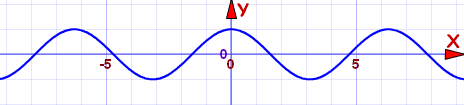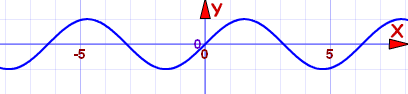# Even and Odd Functions

They are special types of functions

## Even Functions

A function is "even" when:

f(x) = f(−x) for all x

In other words there is symmetry about the y-axis (like a reflection):This is the curve f(x) = x2+1

They got called "even" functions because the functions x2, x4, x6, x8, etc behave like that, but there are other functions that behave like that too, such as cos(x):Cosine function: f(x) = cos(x)
It is an even function

But an even exponent does not always make an even function, for example (x+1)2 is not an even function.

## Odd Functions

A function is "odd" when:

−f(x) = f(−x) for all x

Note the minus in front of f(x): −f(x).

And we get origin symmetry:This is the curve f(x) = x3−x

They got called "odd" because the functions x, x3, x5, x7, etc behave like that, but there are other functions that behave like that, too, such as sin(x):Sine function: f(x) = sin(x)
It is an odd function

But an odd exponent does not always make an odd function, for example x3+1 is not an odd function.

## Neither Odd nor Even

Don't be misled by the names "odd" and "even" ... they are just names ... and a function does not have to be even or odd.

In fact most functions are neither odd nor even. For example, just adding 1 to the curve above gets this:This is the curve f(x) = x3−x+1

It is not an odd function, and it is not an even function either.
It is neither odd nor even

## Even or Odd?

### Example: is f(x) = x/(x2−1) Even or Odd or neither?

Let's see what happens when we substitute −x:

f(−x) = (−x)/((−x)2−1)
=−x/(x2−1)
=−f(x)

So f(−x) = −f(x) , which makes it an Odd Function

## Even and Odd

The only function that is even and odd is f(x) = 0

## Special Properties

• The sum of two even functions is even
• The sum of two odd functions is odd
• The sum of an even and odd function is neither even nor odd (unless one function is zero).

Multiplying:

• The product of two even functions is an even function.
• The product of two odd functions is an even function.
• The product of an even function and an odd function is an odd function.